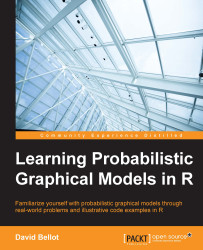•#### Learning Probabilistic Graphical Models in R#### Overview of this book

Probabilistic graphical models (PGM, also known as graphical models) are a marriage between probability theory and graph theory. Generally, PGMs use a graph-based representation. Two branches of graphical representations of distributions are commonly used, namely Bayesian networks and Markov networks. R has many packages to implement graphical models. We’ll start by showing you how to transform a classical statistical model into a modern PGM and then look at how to do exact inference in graphical models. Proceeding, we’ll introduce you to many modern R packages that will help you to perform inference on the models. We will then run a Bayesian linear regression and you’ll see the advantage of going probabilistic when you want to do prediction. Next, you’ll master using R packages and implementing its techniques. Finally, you’ll be presented with machine learning applications that have a direct impact in many fields. Here, we’ll cover clustering and the discovery of hidden information in big data, as well as two important methods, PCA and ICA, to reduce the size of big problems.
Learning Probabilistic Graphical Models in RCreditswww.PacktPub.comPrefaceFree Chapter
Probabilistic ReasoningExact InferenceLearning ParametersBayesian Modeling – Basic ModelsApproximate InferenceBayesian Modeling – Linear ModelsProbabilistic Mixture ModelsAppendixIndex## EM for mixture models

The standard way for fitting mixture models is the EM algorithm or Expectation Maximization. This algorithm was the focus of Chapter 3, Learning Parameters. So here, we just recall the basic principles of this algorithm again, to later show a Bernoulli mixture model.

A good package to use in R is `mixtools` to learn mixture models. A thorough presentation of this package is given in the Journal of Statistical Software, Oct 2009, Vol 32, Issue 6, mixtools: An R Package for Analyzing Finite Mixture Models.

The EM algorithm is a good choice for learning a mixture model. Indeed, in Chapter 3, Learning Parameters, we saw that when data is missing or even when variables are hidden (that is, all their respective data is missing), the EM algorithm will proceeds in two steps: first compute the expected value of the missing variables, so that to do as if the data is fully observed, and then maximize an objective function, usually the likelihood. Then, given the new set of parameters...